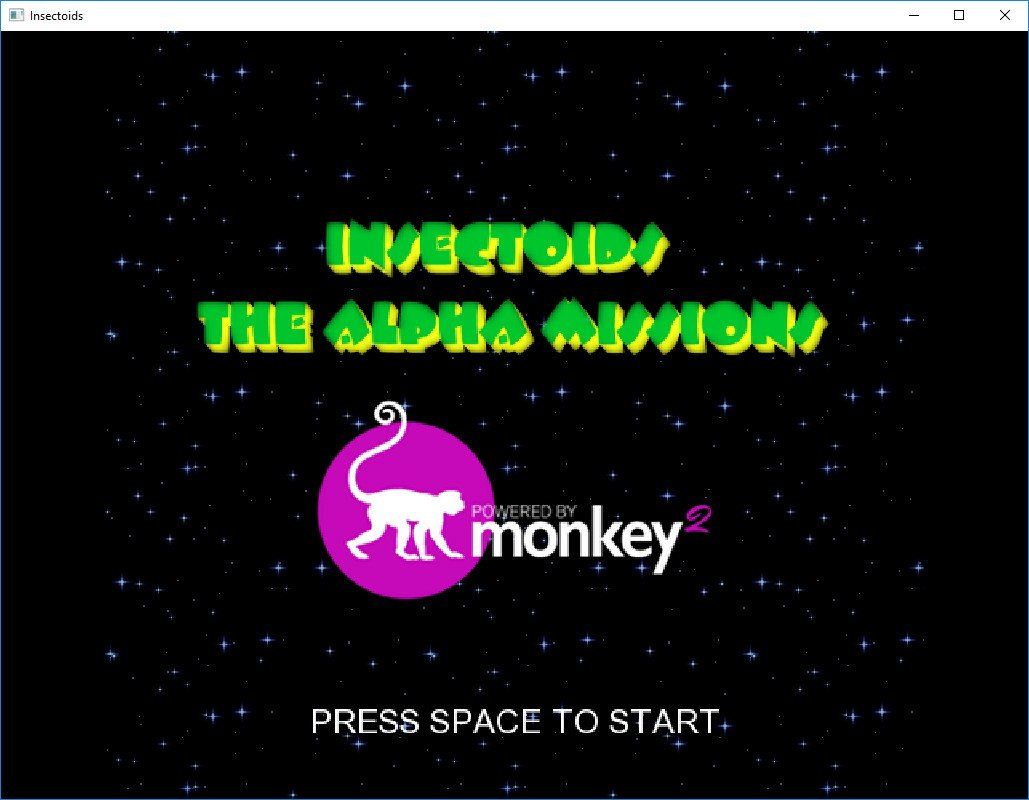-=+=- -=+=- -=+=- -=+=- -=+=- -=+=- -=+=- -=+=- -=+=- -=+=- -=+=- -=+=- -=+=- -=+=- -=+=- -=+=- -=+=- -=+=- -=+=- -=+=- -=+=- -=+=- -=+=- -=+=- -=+=- -=+=- -=+=- -=+=- -=+=- -=+=- (c) WidthPadding Industries 1987 0|663|0 -=+=- -=+=- -=+=- -=+=- -=+=- -=+=- -=+=- -=+=- -=+=- -=+=- -=+=- -=+=- -=+=- -=+=- -=+=- -=+=- -=+=- -=+=- -=+=- -=+=- -=+=- -=+=- -=+=- -=+=- -=+=- -=+=- -=+=- -=+=- -=+=- -=+=-
SoCoder -> Showcase Home -> Shoot 'em Up

therevillsgamesCreated : 23 September 2017
Edited : 25 September 2017
Language : Monkey2

### Insectoids!

Shoot the Bugs!

GitHub - Source Code and Assets
ScreenshotsPort of the classic BlitzPlus Insectoids game coded in Monkey2.

Saturday, 23 September 2017, 04:17
GfKNeeded a break from solitaire?Saturday, 23 September 2017, 04:31
therevillsgamesOH! YES!

I create a few little demos/games between the solitaire games.... keeps me sane, but normally they dont result to much and look pretty crappy!Saturday, 23 September 2017, 22:08
therevillsgamesFixed a couple of bugsMonday, 25 September 2017, 03:02
therevillsgamesCommitted to GitHub:

https://github.com/therevills/InsectoidsFriday, 29 September 2017, 03:33
therevillsgamesRunning in a browser:

https://monkeycoder.co.nz/insectoids/Insectoids.htmlFriday, 29 September 2017, 11:02
PakzAny chance of tablet/phone controls?Sunday, 01 October 2017, 16:45
therevillsgamesI'll look into it...Tuesday, 03 October 2017, 04:21
steve_ancellJust realised that the powered by monkey2 logo in the pic above looks rather like those little b******s in Manic Miner's Sixteenth Cavern.

|update| -=-=- |update|Tuesday, 03 October 2017, 04:46
rockfordIt does indeed!